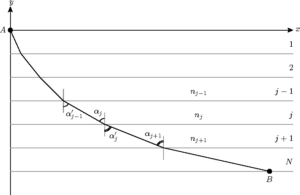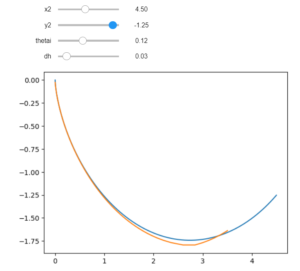# The Brachistochrone and Snell’s Law: A Surprising Connection

Brachistochrone – A word originating from Greece that means “shortest time.” The brachistochrone problem is a well known problem in the world of physics that involves finding a curve such that, assuming there is no friction, a ball traveling along this curve would reach its destination in the shortest possible time. Initially discovered by Johann Bernoulli, a famous Swiss mathematician, the brachistochrone curve has a variety of interesting properties, such as the fact that it is a cycloid, the path mapped out by a point on a circle as it rolls on a flat surface. Additionally, a subsection of the curve also acts as a tautochrone curve, a curve that causes a ball to reach its destination in the same amount of time regardless of where it begins its journey. However, perhaps the most interesting fact about the brachistochrone is that it was initially solved by Bernoulli using Snell’s law, a law that dictates the path traveled by light when moving between different mediums.A cycloid is mapped out in red. When inverted, this curve matches the shape of the brachistochrone.

Initially, it may not be readily obvious why Snell’s law would approximate a brachistochrone curve, but with a bit of physical intuition, it makes perfect sense. First, the reason why Snell’s law works must first be understood. Snell’s law states that the ratio of the sines of the angles formed by the refraction of light when passing through a medium is equal to the ratio of the speed within the two media.

With a bit of calculus not shown here (perhaps in a future article), it can be shown that this equation comes as a consequence of minimizing the time taken for light to travel from one point to another between the two media. This is because light naturally always takes the path of shortest time, and this is what connects Snell’s law to the brachistochrone. When a ball is dropped, it is constantly changing velocity due to gravity, so you can envision a ball as traveling through a continuous set of layers of different velocities. If the ball wanted to travel the path of shortest time, it would follow Snell’s law at every point in its path, so the brachistochrone curve should have the property of having the ratio of instantaneous change in the velocity of the ball match the ratio of the angles formed by a vertical line at that point of the curve.Here, the line travels through several “layers” with different speeds due to gravity and diffracts according to Snell’s law each time.

Of course, it’s one thing to be told that Snell’s law maps out the brachistochrone, but it’s not as satisfying as seeing it for yourself, which is why I coded a simulation in Python to try and match up a curve constructed from Snell’s law with a brachistochrone curve.The top two sliders change the initial conditions of the actual brachistochrone in blue, while the bottom two sliders change the initial angle and the number of layers for the Snell’s law approximation in orange.

For more information on the brachistochrone curve, check out Vsauce or 3Blue1Brown’s videos on the topic below: